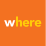# Parse rasters by string in name

691
6
08-12-2018 04:00 PMNew Contributor

Hello! I have 750+ rasters with precipitation data. The folder contains a raster for each month for the years 1945-2009. I need to find the mean of each year in a new raster. However, I am getting caught up on how to parse all the rasters with the specific years in their name. I am only able to run the calcs on one  year at a time. The rasters are named like Oct2009.tif, Nov2009.tif, Nov2008.tif, etc.

``````import arcpy
arcpy.CheckOutExtension("Spatial")
# Define input workspace and create list of rasters
arcpy.env.workspace = r'C:\Users\dzelmanfahm\Desktop\DEM_Trials'
rasters = arcpy.ListRasters("*2009*")
print(rasters)
# Run cell statistics
calc = arcpy.sa.CellStatistics(rasters, statistics_type = "MEAN")
calc.save(r'C:\Users\dzelmanfahm\Desktop\OUTPUT_DEM_TRIALS\Raster.tif')‍‍‍‍‍‍‍‍‍``````
Tags (5)
6 RepliesbyEsri Esteemed Contributor

You can probably use something like this (untested code):

``````import arcpy
import os

# settings
ws_in = r'C:\Users\dzelmanfahm\Desktop\DEM_Trials'
ws_out = r'C:\Users\dzelmanfahm\Desktop\output folder name without spaces'

arcpy.env.workspace = ws_in
ras_names = arcpy.ListRasters()

arcpy.CheckOutExtension("Spatial")

for year in range(1945, 2010):
lst_ras_year = [ras_name for ras_name in ras_names if str(year) in ras_name]
out_name = "mean{}.TIF".format(year)
out_name_path = os.path.join(ws_out, out_name)
mean_ras = arcpy.sa.CellStatistics(lst_ras_year, statistics_type = "MEAN")
mean_ras.save(out_name_path)
‍‍‍‍‍‍‍‍‍‍‍‍‍‍‍‍‍‍``````

It consists of creating a list of all the rasters and simply loop through the years and create a list of the rasters for a single year and use that for the calculation and output namingNew Contributor

I appreciate the help Xander!  It runs, and I was a little overzealous and thought it was exactly what I need. Unfortunately, it doesn't loop through all the years and only outputs the mean of the very first year.byEsri Esteemed Contributor

Interesting... It might be good to include some print statements to be sure that it loops through the years and to help find what is going wrong.

Could you run the code below and post back the message that are printed?

``````import arcpy
import os

# settings
ws_in = r'C:\Users\dzelmanfahm\Desktop\DEM_Trials'
ws_out = r'C:\Users\dzelmanfahm\Desktop\output folder name without spaces'

arcpy.env.workspace = ws_in
ras_names = arcpy.ListRasters()
print(ras_names)

arcpy.CheckOutExtension("Spatial")

for year in range(1945, 2010):
print("\nyear:{}".format(year))
lst_ras_year = [ras_name for ras_name in ras_names if str(year) in ras_name]
print("raster for this year:{}".format(lst_ras_year))
out_name = "mean{}.TIF".format(year)
out_name_path = os.path.join(ws_out, out_name)
print("out_name_path:{}".format(out_name_path))
mean_ras = arcpy.sa.CellStatistics(lst_ras_year, statistics_type = "MEAN")
mean_ras.save(out_name_path)

arcpy.CheckInExtension("Spatial")‍‍‍‍‍‍‍‍‍‍‍‍‍‍‍‍‍‍‍‍‍‍‍‍``````New Contributor

It is still only kicking out the first year (mean1945.tif). The loop stopped at 1946.

My raster list is short at the moment, I used only a few months from the years 1945-1949 in my trial folder.

``````import arcpy
import os

# settings
ws_in = r'C:\Users\dzelmanfahm\Desktop\DEM_Trials'
ws_out = r'C:\Users\dzelmanfahm\Desktop\OUTPUT_DEM_TRIALS'

arcpy.env.workspace = ws_in
ras_names = arcpy.ListRasters()
print(ras_names)

arcpy.CheckOutExtension("Spatial")

for year in range(1945, 1950):
print("\nyear:{}".format(year))
lst_ras_year = [ras_name for ras_name in ras_names if str(year) in ras_name]
print("raster for this year:{}".format(lst_ras_year))
out_name = "mean{}.TIF".format(year)
out_name_path = os.path.join(ws_out, out_name)
print("out_name_path:{}".format(out_name_path))
mean_ras = arcpy.sa.CellStatistics(lst_ras_year, statistics_type = "MEAN")
mean_ras.save(out_name_path)

arcpy.CheckInExtension("Spatial")
['pptApr1947.tif', 'pptApril1946.tif', 'pptDec1946.tif', 'pptDec1948.tif', 'pptFeb1945.tif', 'pptJune1946.tif', 'pptJune1947.tif', 'pptNov1945.tif', 'pptNov1948.tif', 'pptNov1949.tif', 'pptOct1945.tif', 'pptOct1949.tif']

year:1945
raster for this year:['pptFeb1945.tif', 'pptNov1945.tif', 'pptOct1945.tif']
out_name_path:C:\Users\dzelmanfahm\Desktop\OUTPUT_DEM_TRIALS\mean1945.TIF

year:1946
raster for this year:['pptApril1946.tif', 'pptDec1946.tif', 'pptJune1946.tif']
out_name_path:C:\Users\dzelmanfahm\Desktop\OUTPUT_DEM_TRIALS\mean1946.TIF‍‍‍‍‍‍‍‍‍‍‍‍‍‍‍‍‍‍‍‍‍‍‍‍‍‍‍‍‍‍‍‍‍``````

Thank you again!byEsri Esteemed Contributor

Your ras_names list seems OK, and so does the list per year. Only it stops at 1946 when it should run up to 1949. Since you only have the raster for 1945, probably the calculation for 1946 is producing some error. Do you have any error messages? From where do you run the code (stand alone or inside ArcMap or ArcGIS Pro?

Let's try something more:

``````import arcpy
import os

# settings
ws_in = r'C:\Users\dzelmanfahm\Desktop\DEM_Trials'
ws_out = r'C:\Users\dzelmanfahm\Desktop\OUTPUT_DEM_TRIALS'

arcpy.env.workspace = ws_in
arcpy.env.overwriteOutput = True
ras_names = arcpy.ListRasters()
print(ras_names)

arcpy.CheckOutExtension("Spatial")

for year in range(1945, 1950):
print("\nyear:{}".format(year))
lst_ras_year = [ras_name for ras_name in ras_names if str(year) in ras_name]
print("raster for this year:{}".format(lst_ras_year))
out_name = "mean{}.TIF".format(year)
out_name_path = os.path.join(ws_out, out_name)
print("out_name_path:{}".format(out_name_path))
try:
mean_ras = arcpy.sa.CellStatistics(lst_ras_year, statistics_type = "MEAN")
mean_ras.save(out_name_path)
except Exception as e:
print(e)

arcpy.CheckInExtension("Spatial")‍‍‍‍‍‍‍‍‍‍‍‍‍‍‍‍‍‍‍‍‍‍‍‍‍‍‍‍``````

I have put a try except around the Cell Statistics to see if this is the cause for the code to stop before it should and I included the env setting overwriteOutput = True.byMVP Esteemed Contributor

Comes up a lot... another option, just needs a year loop